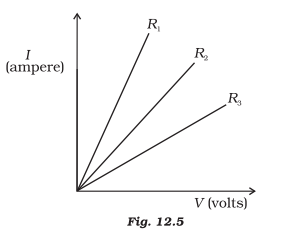# A student carries out an experiment and plots the V-I graph of three samples of nichrome wire with resistances R1, R2 and R3 respectively. Which of the following is true? (a) R1 = R2 = R3 (b) R1 > R2 > R3 (c) R3 > R2 > R1 (d) R2 > R3 > R1Answer: (c) R3 > R2 > R1

Slope for R1 is maximum and slope for R3 is the minimum.

R1 slope > R2 slope > R3 slope

We know that

Resistance of the wire = 1/ Slope of I-V graph

Current flow is inversely proportional to resistance. Highest resistance will show less flow of current.

∴ R3 > R2 > R1(0)(0)